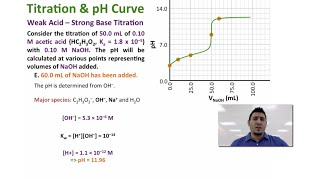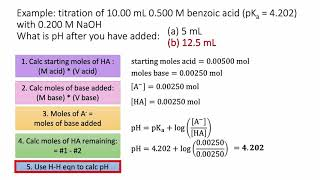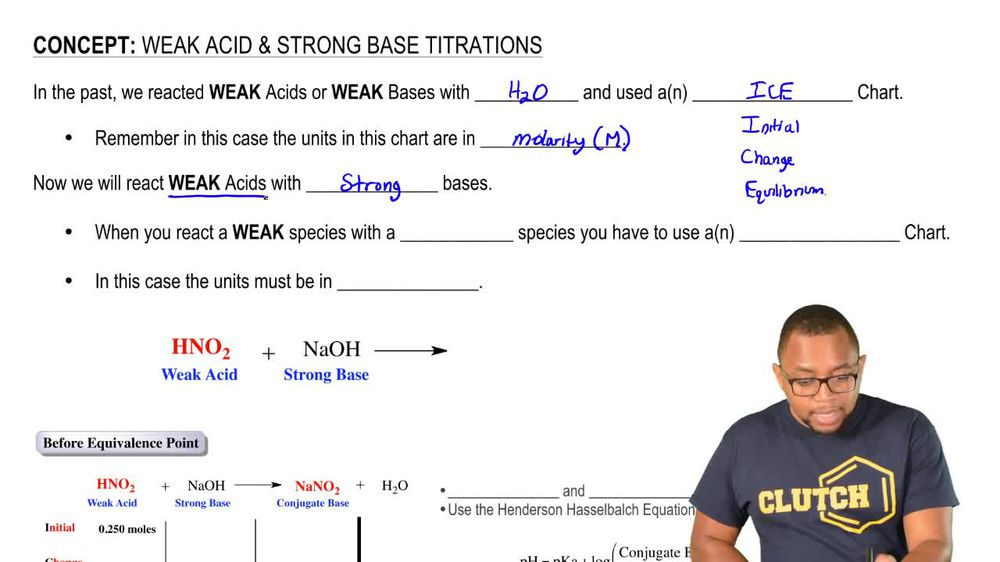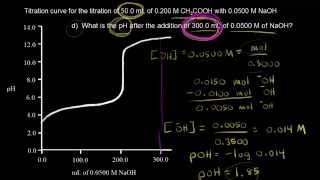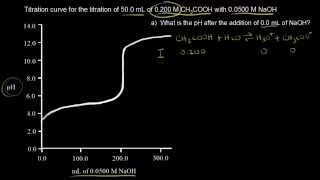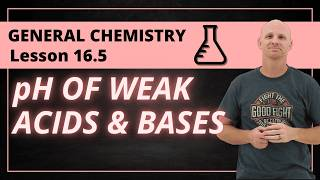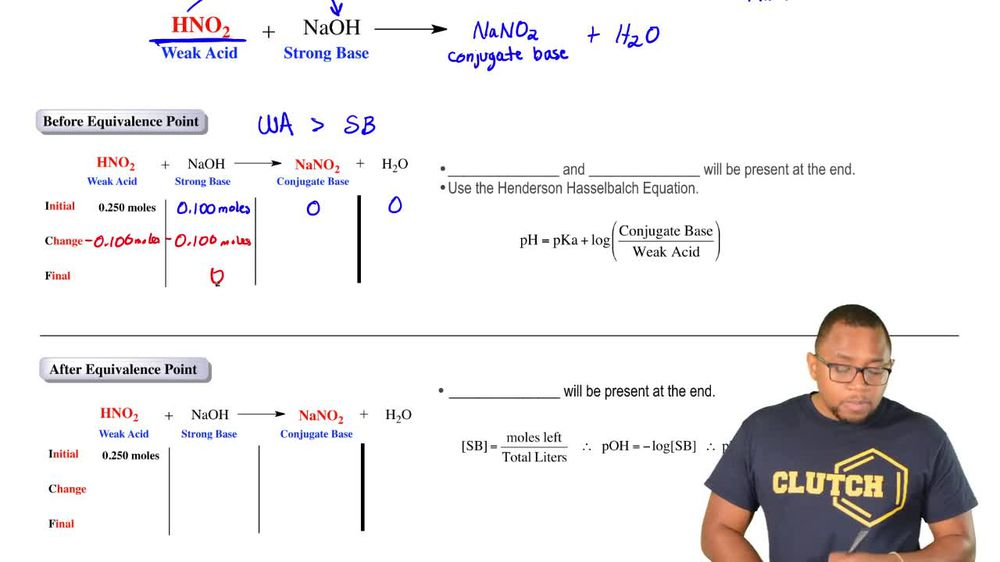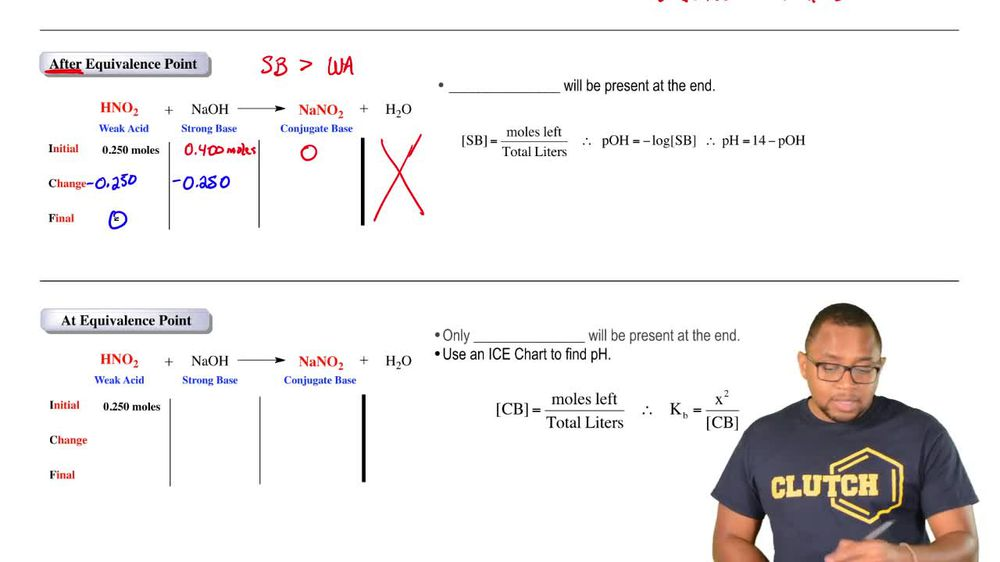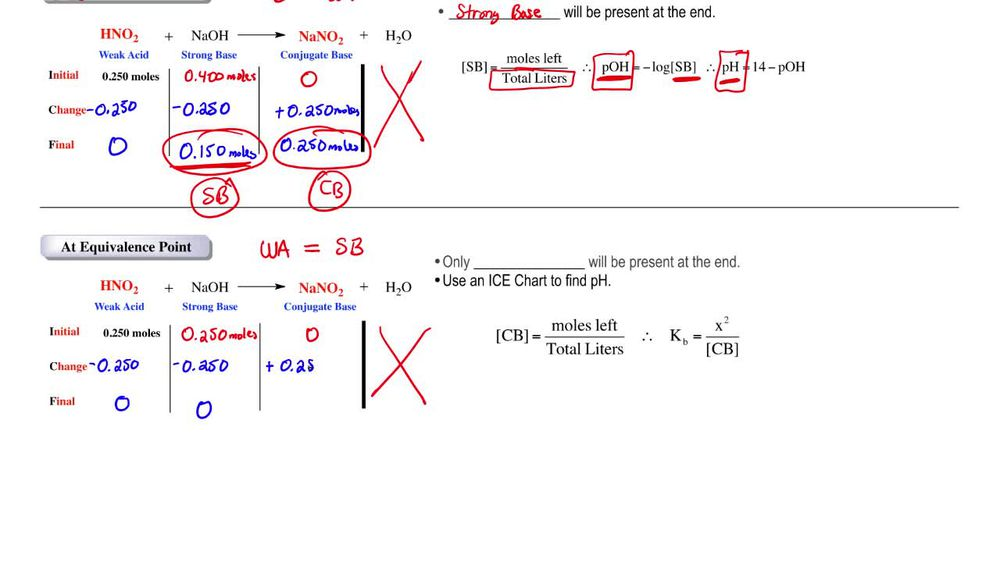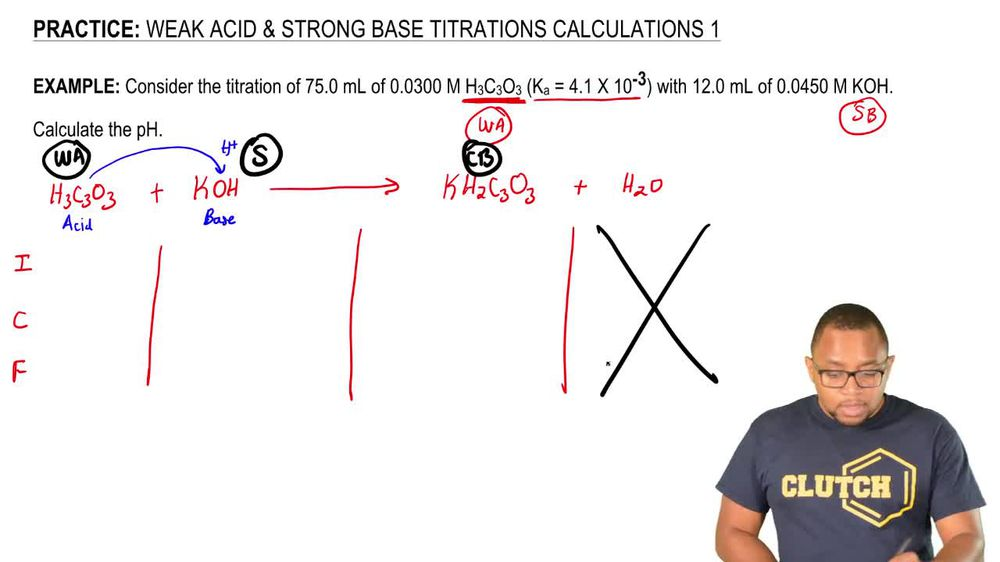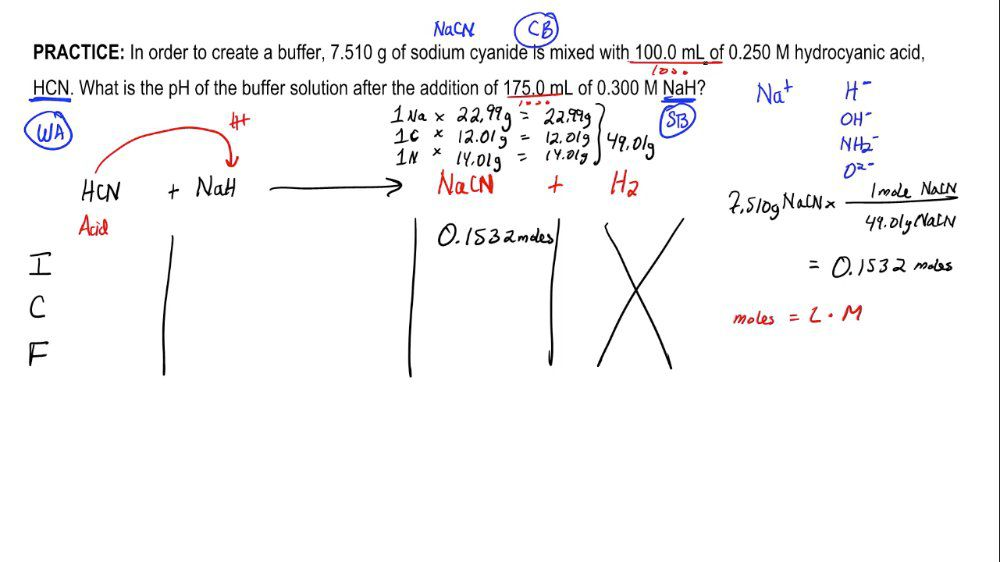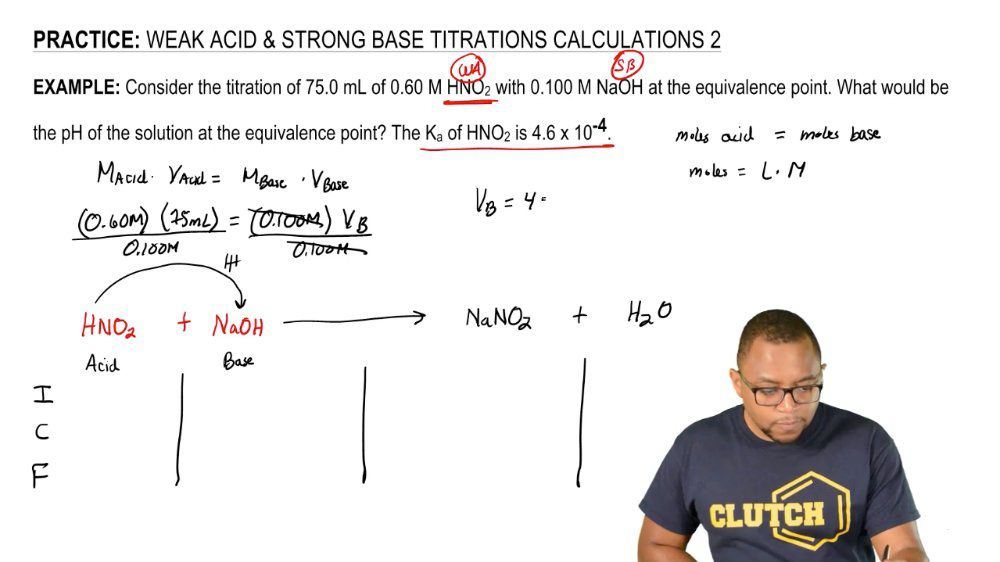Start typing, then use the up and down arrows to select an option from the list.
1. 18. Aqueous Equilibrium2. Weak Acid Strong Base Titrations# Weak Acid Strong Base Titrations 3

by Jules Bruno
113 views
6
0
considered the Thai trey shin of 75 MLS of 750.60 Moeller nitrous acid with 0.100 Mueller sodium hydroxide at the equivalence point will be the pH of the solution at the equivalence point were given. The K of nitrous acid is being 4.6 times 10 to the negative. Four. Now realize here that we have nitrous acid, which is a weak acid. We know it's a weak acid from the rules that we learn about oxy acids. We also know it's a weak acid because it's k A value is less than one. Here it reacts with sodium hydroxide, which is a strong base. So we have a weak species with a strong species reacting with one another. So we know we're gonna have to set up in I C F chart. Now here. We're going to say in our I c F chart, whatever strong has to be a reactant are strong base is going to react with weak acid. Okay, here we have an acid here we have a base. Remember, an acid donates H plus over to the base will produce H 20 and sodium nitrite. We have initial change final. Now remember, in a nice CF chart we have moles is the units. And because we're at the equivalence point, that means that my moles of acid equals my moles of base. Also remember that when it comes to moles, moles equals leaders times molar ity. Because of these two facts weaken say here that m acid times v acid equals and base times v base. So all we're doing here is we're adapting, adapting the dilution equation to help fit our acid and base definitions of being at the equivalence point. So here we're going to say we have 0.60 Moeller of our weak acid, 75 miles of it. We have 100 mol of our strong base. We don't know what the volume of the basis. So we divide both sides now by 0.100 Mueller. So that's gonna give me volume of the base equals 450 mL. Later on, we'll see why it was important to determine this volume for a base for now. Realize again, we're at the equivalence point. So whatever the moles of my acid are, my moles of base would be the same. Remember, we're gonna divide this these MLS here by 1000 and multiply them by the molar ity that will give us our moles of the nitrous acid. So when we do that, we get 0.45 moles again. Remember, we're at the equivalence point. So my moles of base are also 0.0.4 point 045 moles. We have no information on our conjugal based, so it's equal to zero. Remember, in an I c F chart, we only care about three things. The weak acid, the conjugate base and whatever is strong. We ignore this fourth species here. Now we look on the react inside, the smaller molds will subtract from the larger one. But here they're both the same amount of moles. So at the end, they're both going to be zero. Whatever happens on the react inside, the opposite happens on the product side. So we add this amount. At this point, we're gonna say all we have left at the end is conjugate base conjugate base is equal to the toe a week base. And remember, if you have a weak base or weak acid, how do we determine th we determine it with an ice chart. So at the equivalence point is the worst situation. We first have to set up a nice CF chart. Then we're gonna set up a nice chart. Now, remember, in a nice chart, there's a couple things we need to keep in mind. One units are in polarity, and secondly, we're going to ignore the metal off the conjugate base. That's because the metal involved is just a spectator ion. It's a neutral metal ion. So that means for ice chart. We're just gonna We're not gonna work with any, you know, to We're just gonna work with N O to minus. Okay, that's all we're gonna be concerned with. That's what we're gonna bring down into the ice chart. We need the polarity of this continent. Base similarity of the conjugate base will be the moles of it left divided by the total volume. So now we see why we had to determine the volume of our strong base because we need the total volume. So here we're gonna say that the total volume is the 75 MLS of the weak acid and the 450 m els of the strong base. So my total volume equals 0.75 leaders plus 0.450 leaders. All I did is I divided both the MLS by 1000. Okay, so that's my total volume. Take that total volume and plug it here on the bottom to find the polarity of my conjugal base. Okay, so at the end, that's gonna give me 0.857 Moeller of my nitrite ion. Now that we have the mole Arat e, we're gonna set up our ice chart. So remember, in a nice chart, are weak acid in our week based react with water. We have double arrows again because it's a week species water. He'll act as the acid. And this is my base, remember? And acid donates h plus, so we'll get H and l tube plus O H minus. We're dealing with initial change equilibrium. So remember, in a nice chart, we ignore water because the liquid our initial concentration, what we calculated is 0.857 Mueller products initially are both zero. We lose reactant in order to make products. Now we're dealing with the conjugate base. Remember, it's a week base week basis. Don't use K they use K B. So the K were given in the beginning, we're gonna have to change that two K b. So there's a lot of work involved in this. So we're gonna say, Remember K A Times K B equals K W. Plug in the number for K. Remember, kW is 1.0 times 10 to the negative 14. Divide both sides here by K A. So we'll get KB equal to 2.17 times 10 to the negative 11. So that's our KB that we're going to use. K B is equal to products. Overreact INTs, so it's equal to X squared over 0.857 minus X. Remember, we can use the 5% approximation method to see if we could ignore this minus X. So with the 5% approximation method, we're gonna take the initial concentration and divided by R K B. And if we get a value greater than 500 we can ignore that minus X. So the initial concentration of my week base is 0.857 Moeller, divided by the K B. We just calculated as 2.17 times 10 to the negative 11. That gives me 3.94 times 10 to the ninth. That number is much greater than 500. So that means we can ignore this minus X here, so we can drop this minus X and just solve for X now. So our K B look at our baby here, plug in the value that we determined we're gonna multiply both sides by 0. So that's gonna give you my X squared X squared equals 1.860 times 10 to the negative 12. Take the square root of both sides here. X equals 1.364 times 10 to the negative. Six Mohler. Now, remember, anytime we find X, that's gonna give us either H 30 plus or O H minus. If we look at our ice chart, the X that we just found is connected. Toa are h minus. Okay, so it's equal toe o h minus. So we're gonna say now p o. H equals negative Log of O. H minus concentration plug in the number that we just got. Okay, when we do that, it's going to give us our r p H So that's going to be r P O H 5. Because we know our p o h. We know our pH so pH equals 14 minus P o. H. So that comes out to 8. so we can see that there's a lot of work that goes into determining the pH at the equivalence point. Whenever we titrate a week species with a strong species again just to recap everything we went through, we realize here we're dealing with a weak acid in a strong base. So we know we need to set up a nice CF chart because we're at the equivalence point. They have equal moles. That means they're going to totally destroy each other in the reaction. And all we'll have left is conjugate base. Conjugate base is the same thing as weak base. So to determine its pH, we have to set up a nice chart. Once we set up the ice chart, we'll figure out what the concentration of O. H minus is. From that you can figure out your P o. H. And from there are pH. So keep in mind the steps necessary to determine the ph when a weak acid in strong base are reacting at the equivalents point, we set up a nice CF chart, then a nice chart.